World Statistics Day was yesterday, but we’re celebrating all week long! This celebration means acknowledging the impact statistics has on our world. Who is your favorite statistician? Share with us who they are and why they top your favorites list.
Choose Language Hide Translation Bar
Highlighted

## Plotting first column versus any column with Ic in the title

Any help is very much appreciated!

5 REPLIES 5
Highlighted

## Re: Plotting first column versus any column with Ic in the title

The JMP data table you attached to your discussion is not usable for plotting.  Was the solution to making this data table that was given in your previous discussion, "Running average by data series using For Loop and same script on multiple columns", not the correct solution?  Is there a reason you are continuing to distribute this version of the data table?

If you use the corrected version of the data table, you can take it and go directly to the Fit Y by X platform

Analyze==>Fit Y by X

and select the H(Oe) and place it into the Y Response area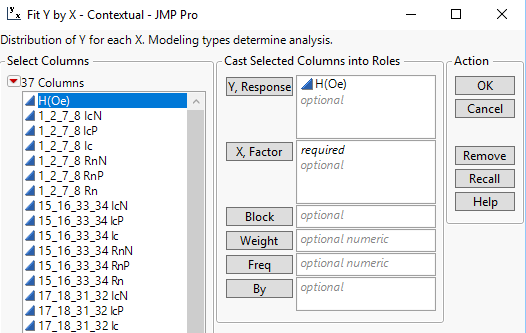Then open the filter for the Select Columns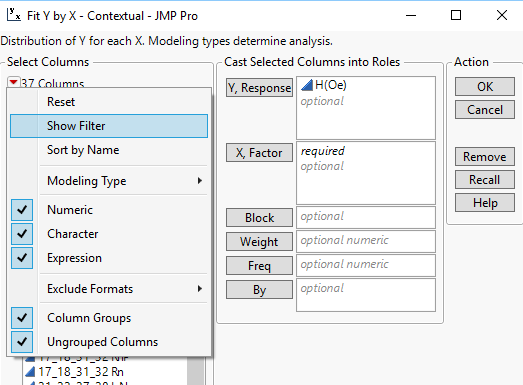Next type in "Ic" into the filter box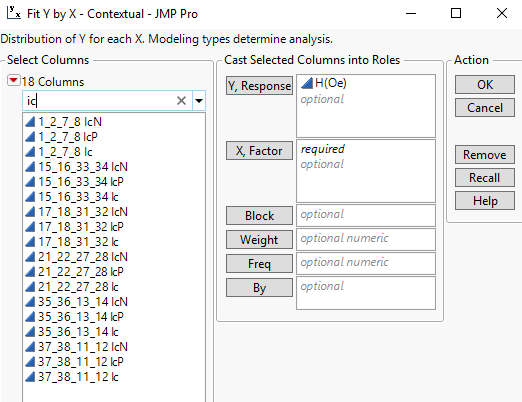Select and place all or however many of them you want to graph into the X Factor selection box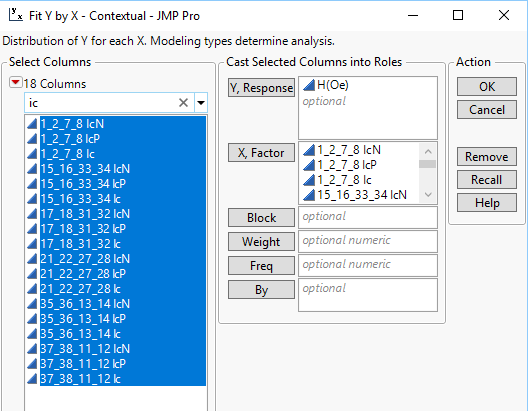Click on OK and all of the graphs will be produced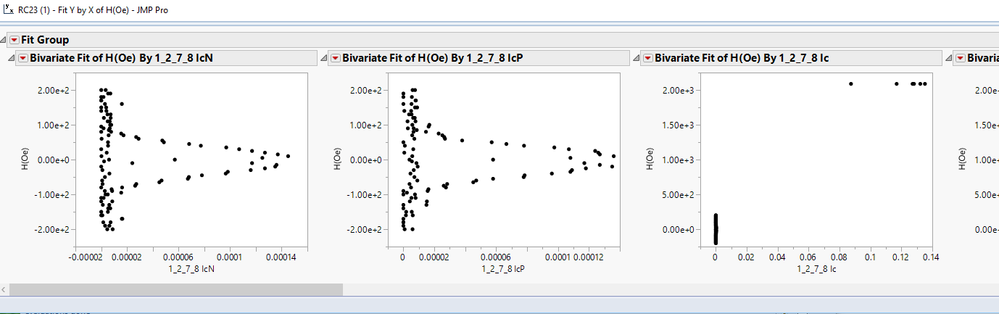If you want the Ic columns to be on the Y axis, just place the H(Oe) column in the X selection box, and all of the Ic columns into the Y selection box

Here is the code I previously provided to convert your attached data table into a usable table

``````Names Default To Here( 1 );
dt = Current Data Table();

// Correct the data table using the JMP table imported from the Excel file
For( i = 2, i <= N Cols( dt ), i++,
Column( dt, i ) << set name(
Word( 1, Column( dt, i ) << get name, " " ) || " " || Column( dt, i )
)
);

dt << delete rows( 1 );

For( i = 2, i <= N Cols( dt ), i++,
Column( dt, i ) << data type( numeric ) << modeling type( continuous )
);

// Change the name of the first column to H(Oe)
Column( dt, 1 ) << set name( "H(Oe)" );``````
Jim
Highlighted

## Re: Plotting first column versus any column with Ic in the title

Thanks again for the help! Is there a simple way to do this with a script?

@txnelson wrote:

The JMP data table you attached to your discussion is not usable for plotting.  Was the solution to making this data table that was given in your previous discussion, "Running average by data series using For Loop and same script on multiple columns", not the correct solution?  Is there a reason you are continuing to distribute this version of the data table?

If you use the corrected version of the data table, you can take it and go directly to the Fit Y by X platform

Analyze==>Fit Y by X

and select the H(Oe) and place it into the Y Response areaThen open the filter for the Select ColumnsNext type in "Ic" into the filter boxSelect and place all or however many of them you want to graph into the X Factor selection boxClick on OK and all of the graphs will be producedIf you want the Ic columns to be on the Y axis, just place the H(Oe) column in the X selection box, and all of the Ic columns into the Y selection box

Here is the code I previously provided to convert your attached data table into a usable table

``````Names Default To Here( 1 );
dt = Current Data Table();

// Correct the data table using the JMP table imported from the Excel file
For( i = 2, i <= N Cols( dt ), i++,
Column( dt, i ) << set name(
Word( 1, Column( dt, i ) << get name, " " ) || " " || Column( dt, i )
)
);

dt << delete rows( 1 );

For( i = 2, i <= N Cols( dt ), i++,
Column( dt, i ) << data type( numeric ) << modeling type( continuous )
);

// Change the name of the first column to H(Oe)
Column( dt, 1 ) << set name( "H(Oe)" );``````

Highlighted

## Re: Plotting first column versus any column with Ic in the title

In my last response, I asked some questions of you concerning the data table you had attached.  The answers to these questions are important, since the purpose of this Community Discusssion group is not to provide a pool of JMP scriptor to generate code, but rather a pool of experienced JMP users to help users with their issues to better understand how to solve them with JMP.  The questions I asked were an attempt to have you feedback that what was provided to you, you understand what was given to you.

I will answer the current resquest, assuming you have studied the last response, and understand it.

Below is a simple script that will find all columns in your data table that has "Ic" in it's name.

The code necessary to generate the graphs

``````Names Default To Here( 1 );
dt = Current Data Table();

// Create a list of all numeric column names
colNamesList = dt << get column names( numeric );

// get rid of all names that do not have an "Ic" in it
For( i = N Items( colNamesList ), i >= 1, i--,
If( Contains( Uppercase( ColNamesList[i] ), "IC" ) == 0,
colNamesList = Remove( colNamesList, i, 1 )
)
);

// You now have a list of columns that each column has "Ic" in it
// So you can now use it in any platform code you put after this point
// in the script``````

To generate the code necessary to run the Fit Y by X platform, as illustrated in my previous response, all that has to be done, is to tell JMP to Save Script.  And, it will generate a script that will reproduce the output just as it exists.  So if this is the output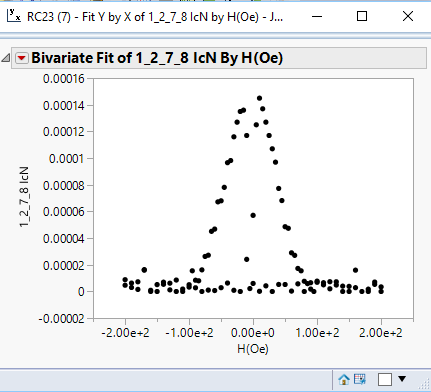You can click on the red triangle and choose the option to save the script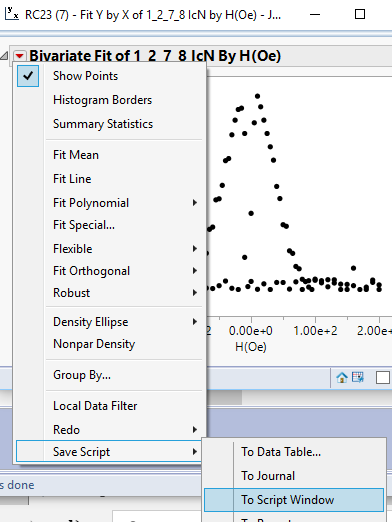and you will get the script

``Bivariate( Y( :Name( "1_2_7_8 IcN" ) ), X( :Name( "H(Oe)" ) ) );``

So, if you add this line of JSL to the above script and run it, you will get the graph generated in your script.  But, it will only generate the one chart.  So taking a bit of code from your question on this topic called "

##### Running average by data series using For Loop and same script on multiple columns", you can substitute into the code
``Eval( colNamesList )``

where the Y column selection is:

``Bivariate( Y( Eval( colNamesList ) ), X( :Name( "H(Oe)" ) ) );``

and now all graphs will be generated when you run the script. This will solve your question in this discussion, but you have asked one further question, concerning the fitting of a nonlinear.  Can you please provide more details??  Do you want JMP to generate the equation, or do you have the equation?   Is this a polynomial fit, or is it generated using nonlinear fitting methodology? Do you want it fit onto the graphs being produced by the above scripts, or are you asking a completly diffierent question?

Jim
Highlighted

## Re: Plotting first column versus any column with Ic in the title

Oh, okay i read over your last response and guess I've been really rushed to get this figured out by the end of the week that I brushed over the heart of your question. I am reading through the scripting guide and figuring these things out, I guess I didn't realize that when you save to script it provides all the answers you really need. So I will use that tool more often and apologize. And appreciate the continual help and feedback. I wll try to make my questions more focused on the type of problems the community is centered around addressing.

Highlighted

## Re: Plotting first column versus any column with Ic in the title

And do you know a good manner to fit a nonlinear distribution with an equation using a script?

Article Labels

There are no labels assigned to this post.